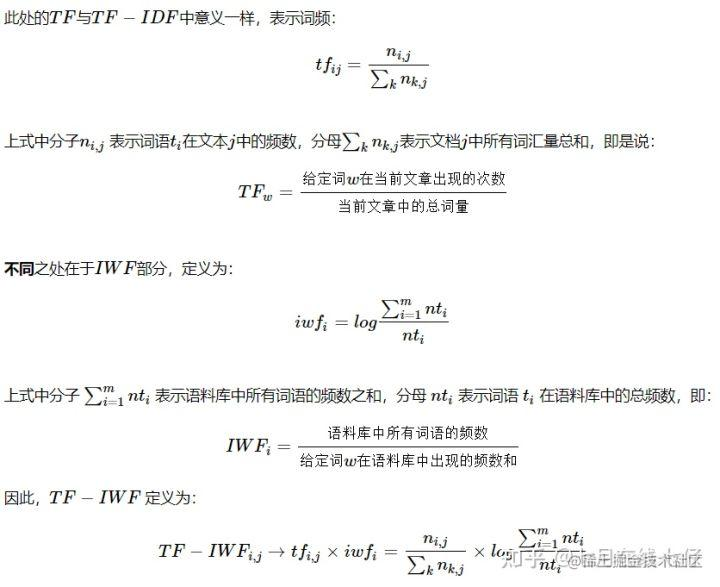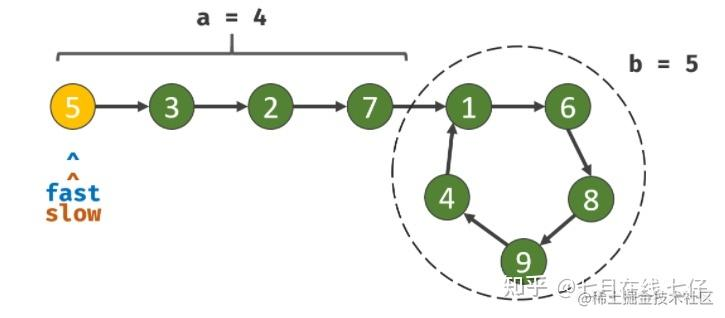# 2021年6月9日，CVTE NLP算法岗面试题9道

## 1.讲一下改进的tf-idf

TF-IDF中的IDF是一种试图抑制噪声的加权，单纯的以为文本频率小的单词就越重要，文本频率越大的单词就越无用，这一方式会在同类语料库中存在巨大弊端，一些同类文本的关键词容易被掩盖。例如：语料库 D 中教育类文章偏多，而文本 j 是一篇属于教育类的文化在那个，那么教育类相关词语的IDF值就会较小，使提取本文关键词的召回率更低。## 3.蒸馏的思想，为什么要蒸馏？

• 推断速度慢
• 对部署资源要求高(内存，显存等)，在部署时，我们对延迟以及计算资源都有着严格的限制。

## 4.有哪些蒸馏方式? 蒸馏中的学生模型是？

Logit Distillation

Beyond Logit Distillation：TinyBert

Curriculum Distillation：

Dynamic Early Exit：FastBert。

## 5.python在内存上做了哪些优化？

python通过内存池来减少内存碎片化，提高执行效率。主要通过引用计数来完成垃圾回收，通过标记-清除解决容器对象循环引用造成的问题，通过分代回收提高垃圾回收的效率。

## 6.怎么节省内存？

• 手动回收不需要用的变量；
• 将数值型数据转化为32位或16位（对数据类型进行限制）

``````def reduce_mem_usage(props):
# 计算当前内存
start_mem_usg = props.memory_usage().sum() / 1024 ** 2
print("Memory usage of the dataframe is :", start_mem_usg, "MB")

# 哪些列包含空值，空值用-999填充。why：因为np.nan当做float处理
NAlist = []
for col in props.columns:
# 这里只过滤了object格式，如果你的代码中还包含其他类型，请一并过滤
if (props[col].dtypes != object):

print("**************************")
print("columns: ", col)
print("dtype before", props[col].dtype)

# 判断是否是int类型
isInt = False
mmax = props[col].max()
mmin = props[col].min()

# Integer does not support NA, therefore Na needs to be filled
if not np.isfinite(props[col]).all():
NAlist.append(col)
props[col].fillna(-999, inplace=True) # 用-999填充

# test if column can be converted to an integer
asint = props[col].fillna(0).astype(np.int64)
result = np.fabs(props[col] - asint)
result = result.sum()
if result < 0.01: # 绝对误差和小于0.01认为可以转换的，要根据task修改
isInt = True

# make interger / unsigned Integer datatypes
if isInt:
if mmin >= 0: # 最小值大于0，转换成无符号整型
if mmax <= 255:
props[col] = props[col].astype(np.uint8)
elif mmax <= 65535:
props[col] = props[col].astype(np.uint16)
elif mmax <= 4294967295:
props[col] = props[col].astype(np.uint32)
else:
props[col] = props[col].astype(np.uint64)
else: # 转换成有符号整型
if mmin > np.iinfo(np.int8).min and mmax < np.iinfo(np.int8).max:
props[col] = props[col].astype(np.int8)
elif mmin > np.iinfo(np.int16).min and mmax < np.iinfo(np.int16).max:
props[col] = props[col].astype(np.int16)
elif mmin > np.iinfo(np.int32).min and mmax < np.iinfo(np.int32).max:
props[col] = props[col].astype(np.int32)
elif mmin > np.iinfo(np.int64).min and mmax < np.iinfo(np.int64).max:
props[col] = props[col].astype(np.int64)
else: # 注意：这里对于float都转换成float16，需要根据你的情况自己更改
props[col] = props[col].astype(np.float16)

print("dtype after", props[col].dtype)
print("********************************")
print("___MEMORY USAGE AFTER COMPLETION:___")
mem_usg = props.memory_usage().sum() / 1024**2
print("Memory usage is: ",mem_usg," MB")
print("This is ",100*mem_usg/start_mem_usg,"% of the initial size")

## 7.pandas库怎么读取超大型文件？

``````data_path = r'E:\python\Study\BiGData\demo.csv'
# 分块，每一块是一个chunk，之后将chunk进行拼接
df = pd.read_csv(path, engine='python', encoding='gbk', iterator=True)
loop = True
chunkSize = 10000
chunks = []
while loop:
try:
chunk = df.get_chunk(chunkSize)
chunks.append(chunk)
except StopIteration:
loop = False
print("Iteration is stopped.")
df = pd.concat(chunks, ignore_index=True)

## 8.无重复字符的最长子串

start 初始为0，用end线性遍历每个字符，用 recod 记录下每个字母最新出现的下标

``````class Solution:
def lengthOfLongestSubstring(self, s: str) -> int:
record = {}
start,res = 0,0
for end in range(len(s)):
if s[end] in record:
start = max(start, record[s[end]] + 1)
record[s[end]] = end
res = max(res, end - start + 1)
return res

## 9.判断链表是否有环、链表环的入口

``````class Solution:
def hasCycle(self, head: ListNode) -> bool:
seen = set()
return True
return False

``````class Solution:
def hasCycle(self, head: ListNode) -> bool:
while fast and fast.next:
slow = slow.next
fast = fast.next.next
if fast == slow:
return True
return False代码如下：

``````class Solution:
def detectCycle(self, head: ListNode) -> ListNode:
while True:
if not (fast and fast.next):return
fast = fast.next.next
slow = slow.next
if fast == slow:break
while fast != slow:
fast = fast.next
slow = slow.next
return fast